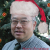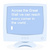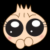# Java中怎么将大写中文金额(壹万伍仟肆佰壹拾元贰角捌分肆厘)转换成数字

1``````import java.util.*;
/**
* 中文數字转阿拉伯数组【十万九千零六十  --> 109060】
* @author 雪见烟寒
* @param chineseNumber
* @return
*/

public class Chinese2Arabian{

@SuppressWarnings("unused")
private static int chineseNumber2Int(String chineseNumber){
int result = 0;
int temp = 1;//存放一个单位的数字如：十万
int count = 0;//判断是否有chArr
char[] cnArr = new char[]{'壹', '贰', '叁', '肆', '伍', '陆', '柒', '捌', '玖'};
char[] chArr = new char[]{'拾', '佰', '仟', '万', '亿'};
for (int i = 0; i < chineseNumber.length(); i++) {
boolean b = true;//判断是否是chArr
char c = chineseNumber.charAt(i);
for (int j = 0; j < cnArr.length; j++) {//非单位，即数字
if (c == cnArr[j]) {
if(0 != count){//添加下一个单位之前，先把上一个单位值添加到结果中
result += temp;
temp = 1;
count = 0;
}
// 下标+1，就是对应的值
temp = j + 1;
b = false;
break;
}
}
if(b){//单位{'十','百','千','万','亿'}
for (int j = 0; j < chArr.length; j++) {
if (c == chArr[j]) {
switch (j) {
case 0:
temp *= 10;
break;
case 1:
temp *= 100;
break;
case 2:
temp *= 1000;
break;
case 3:
temp *= 10000;
break;
case 4:
temp *= 100000000;
break;
default:
break;
}
count++;
}
}
}
if (i == chineseNumber.length() - 1) {//遍历到最后一个字符
result += temp;
}
}
return result;
}
public static void main(String args[]){
String s = "壹万伍仟肆佰壹拾";
System.out.println( s + " = " + chineseNumber2Int(s));

}
}``````

``壹万伍仟肆佰壹拾 = 15410``

0``````package net.sealin.helloworld.util;

import java.math.BigDecimal;
import java.math.RoundingMode;
import java.util.Arrays;
import java.util.Comparator;
import java.util.HashMap;
import java.util.List;
import java.util.Map;
import java.util.Objects;
import java.util.Optional;
import java.util.stream.Stream;

/**
* 大写中文转阿拉伯数字
*
* @author Sealin
* @date 2018-10-11
*/

public class Chinese2Arabian {
public enum CurrencyUnit {
YUAN(1, "元", 1),
CORNER(2, "角", 10),
POINT(3, "分", 100),
MIN(4, "厘", 1000);
public final Integer UNIT;
public final String COMMENT;
public final Integer SCALE;

CurrencyUnit(Integer unit, String comment, Integer scale) {
UNIT = unit;
COMMENT = comment;
SCALE = scale;
}

public static Optional<CurrencyUnit> getUnit(String s) {
return Arrays.stream(CurrencyUnit.values()).filter(c -> c.COMMENT.equals(s)).findFirst();
}
}

/**
* @param chineseNumber 大写数字
* @return Double
*/
private static Double chinese2double(String chineseNumber) {
Map<String, String> baseNumber = new HashMap<>(2);
baseNumber.put("零", "0");
baseNumber.put("壹", "1");
baseNumber.put("贰", "2");
baseNumber.put("叁", "3");
baseNumber.put("肆", "4");
baseNumber.put("伍", "5");
baseNumber.put("陆", "6");
baseNumber.put("柒", "7");
baseNumber.put("捌", "8");
baseNumber.put("玖", "9");

Map<String, String> units = new HashMap<>(2);
units.put("拾", "10");
units.put("佰", "100");
units.put("仟", "1000");
units.put("万", "10000");
units.put("亿", "100000000");

String[] chars = chineseNumber.split("");
StringBuilder sb = new StringBuilder();
List<String> chineseList = Arrays.asList(chars);
chineseList.stream().filter(baseNumber.keySet()::contains).forEach(k -> sb.append(baseNumber.get(k)));
CurrencyUnit currencyUnit = chineseList.parallelStream().map(CurrencyUnit::getUnit).filter(
Optional::isPresent).map(Optional::get).max(Comparator.comparing(c -> c.UNIT)).orElse(
CurrencyUnit.YUAN);
Optional<String> first = Stream.of("元整", "元正", "圆整", "圆正").filter(chineseNumber::contains).findFirst();
BigDecimal temp = new BigDecimal(sb.toString());
BigDecimal unitMulti = first.map(s -> {
String[] lastUnit = chineseNumber.substring(chineseNumber.indexOf(s) - 2, chineseNumber.indexOf(s)).split(
"");
return Arrays.stream(lastUnit).map(units::get).filter(Objects::nonNull).map(BigDecimal::new).reduce(
BigDecimal::multiply).map(b -> b.setScale(2, 4)).orElse(new BigDecimal(1));
}).orElse(new BigDecimal(1));
BigDecimal result = temp.multiply(unitMulti);
return result.divide(new BigDecimal(currencyUnit.SCALE), 2, RoundingMode.HALF_UP).doubleValue();
}

public static void main(String[] args) {
System.out.println(chinese2double("壹万伍仟肆佰壹拾元贰角捌分肆厘"));
System.out.println(chinese2double("壹万元整"));
System.out.println(chinese2double("壹拾万元整"));
System.out.println(chinese2double("玖佰玖拾玖万伍仟肆佰元整"));
System.out.println(chinese2double("肆佰元整"));
System.out.println(chinese2double("伍仟元整"));
}
}``````0

0

0雪见烟寒 修改而成的半成品 2：

``````import java.util.*;

public class Chinese2Arabian{

@SuppressWarnings("unused")
private static double chineseNumber2Int(String chineseNumber){
double result = 0;
double temp = 1;//存放一个单位的数字如：十万
int count = 0;//判断是否有chArr
char[] cnArr = new char[]{'壹', '贰', '叁', '肆', '伍', '陆', '柒', '捌', '玖'};
char[] chArr = new char[]{'拾', '佰', '仟', '万', '亿', '角','分','厘'};
for (int i = 0; i < chineseNumber.length(); i++) {
boolean b = true;//判断是否是chArr
char c = chineseNumber.charAt(i);
for (int j = 0; j < cnArr.length; j++) {//非单位，即数字
if (c == cnArr[j]) {
if(0 != count){//添加下一个单位之前，先把上一个单位值添加到结果中
result += temp;
temp = 1;
count = 0;
}
// 下标+1，就是对应的值
temp = j + 1;
b = false;
break;
}
}
if(b){//单位{'十','百','千','万','亿'}
for (int j = 0; j < chArr.length; j++) {
if (c == chArr[j]) {
switch (j) {
case 0:
temp *= 10;
break;
case 1:
temp *= 100;
break;
case 2:
temp *= 1000;
break;
case 3:
temp *= 10000;
break;
case 4:
temp *= 100000000;
break;
case 5:
temp *= 0.1;
break;
case 6:
temp *= 0.01;
break;
case 7:
temp *= 0.001;
break;
default:
break;
}
count++;
}
}
}
if (i == chineseNumber.length() - 1) {//遍历到最后一个字符
result += temp;
}
}
return result;
}
public static void main(String args[]){
String s1 = "壹万伍仟肆佰壹拾圆叁角伍分肆厘";
String s2 = "捌万陆仟肆佰壹拾圆整";
String s3 =  "壹万伍仟肆佰壹拾元贰角捌分肆厘";
String s4 =  "拾壹亿壹仟万伍仟肆佰壹拾元贰角捌分肆厘";
System.out.printf("%s = %6.3f\n",s1,chineseNumber2Int(s1));
System.out.printf("%s = %6.3f\n",s2,chineseNumber2Int(s2));
System.out.printf("%s = %6.3f\n",s3,chineseNumber2Int(s3));
System.out.printf("%s = %6.3f\n",s4,chineseNumber2Int(s4));
}
}``````

``````壹万伍仟肆佰壹拾圆叁角伍分肆厘 = 15410.354

0#### 引用来自“tcxu”的评论

雪见烟寒 修改而成的半成品 2：

``````import java.util.*;

public class Chinese2Arabian{

@SuppressWarnings("unused")
private static double chineseNumber2Int(String chineseNumber){
double result = 0;
double temp = 1;//存放一个单位的数字如：十万
int count = 0;//判断是否有chArr
char[] cnArr = new char[]{'壹', '贰', '叁', '肆', '伍', '陆', '柒', '捌', '玖'};
char[] chArr = new char[]{'拾', '佰', '仟', '万', '亿', '角','分','厘'};
for (int i = 0; i < chineseNumber.length(); i++) {
boolean b = true;//判断是否是chArr
char c = chineseNumber.charAt(i);
for (int j = 0; j < cnArr.length; j++) {//非单位，即数字
if (c == cnArr[j]) {
if(0 != count){//添加下一个单位之前，先把上一个单位值添加到结果中
result += temp;
temp = 1;
count = 0;
}
// 下标+1，就是对应的值
temp = j + 1;
b = false;
break;
}
}
if(b){//单位{'十','百','千','万','亿'}
for (int j = 0; j < chArr.length; j++) {
if (c == chArr[j]) {
switch (j) {
case 0:
temp *= 10;
break;
case 1:
temp *= 100;
break;
case 2:
temp *= 1000;
break;
case 3:
temp *= 10000;
break;
case 4:
temp *= 100000000;
break;
case 5:
temp *= 0.1;
break;
case 6:
temp *= 0.01;
break;
case 7:
temp *= 0.001;
break;
default:
break;
}
count++;
}
}
}
if (i == chineseNumber.length() - 1) {//遍历到最后一个字符
result += temp;
}
}
return result;
}
public static void main(String args[]){
String s1 = "壹万伍仟肆佰壹拾圆叁角伍分肆厘";
String s2 = "捌万陆仟肆佰壹拾圆整";
String s3 =  "壹万伍仟肆佰壹拾元贰角捌分肆厘";
String s4 =  "拾壹亿壹仟万伍仟肆佰壹拾元贰角捌分肆厘";
System.out.printf("%s = %6.3f\n",s1,chineseNumber2Int(s1));
System.out.printf("%s = %6.3f\n",s2,chineseNumber2Int(s2));
System.out.printf("%s = %6.3f\n",s3,chineseNumber2Int(s3));
System.out.printf("%s = %6.3f\n",s4,chineseNumber2Int(s4));
}
}``````

``````壹万伍仟肆佰壹拾圆叁角伍分肆厘 = 15410.354